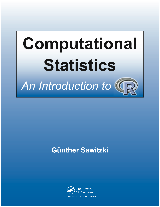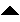Next: What is R? Up: Computational Statistics An Introduction Previous: Contents   Contents

# Introduction

Private note:
This course is an invitation to statistics. It is designed to be compact and comprehensive, so that it can be covered in a short time. But there is more to statistics than you can catch in a short course. If this course gives you some basic ideas, and some tools how to translate statistical concepts into practical computations, it has served its purpose, and you are invited to follow up with an in depth statistical course.

This introduction to R is intended as course material to be used in a compact course or for self-instruction. The course is for students with basic knowledge in stochastics. Notions like distribution function, quantile, expected value and variance are presupposed, as well as some familiarity with statistical features corresponding to these notions. Only a condensed summary is included here. Classical distributions, such as binomial, uniform, and Gaussian should be familiar, together with derived distributions and their asymptotic behaviour. It is not required that the reader has knowledge in statistics properly. But this knowledge is not taught in this course, on the other hand. This course concentrates on the ``computing'' aspects. Statistical concepts and statistical points of view are introduced and discussed. For an in depth discussion, the reader is referred to statistics courses.

A working knowledge in computer usage is presupposed, at least rudimentary knowledge of programming concepts like variables, loops, functions. No extended knowledge in computing is required.

SubsectionsNext: What is R? Up: Computational Statistics An Introduction   Contents
gs 2008-08-23